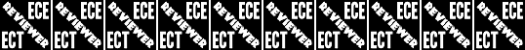# ECT002

20 Questions | Total Attempts: 14172SettingsPriority Level: High (ECE & ECT)

Related Topics
• 1.
What do the symbols on an electrical circuit schematic diagram represent?
• A.

Electrical components

• B.

Logic states

• C.

Digital codes

• D.

Traffic nodes

• 2.
Which of the following is accurately represented in electrical circuit schematic diagrams?
• A.

Wire lengths

• B.

Physical appearance of components

• C.

The way components are interconnected

• D.

All of these choices are correct

• 3.
Which of the following devices or circuits changes an alternating current into a varying direct current signal?
• A.

Transformer

• B.

Rectifier

• C.

Amplifier

• D.

Reflector

• 4.
What best describes a relay?
• A.

A switch controlled by an electromagnet

• B.

A current controlled amplifier

• C.

An optical sensor

• D.

A pass transistor

• 5.
Which of the following can be used to display signal strength on a numeric scale?
• A.

Potentiometer

• B.

Transistor

• C.

Meter

• D.

Relay

• 6.
What component is commonly used to change 120V AC house current to a lower AC voltage for other uses?
• A.

Variable capacitor

• B.

Transformer

• C.

Transistor

• D.

Diode

• 7.
Which of the following is commonly used as a visual indicator?
• A.

LED

• B.

FET

• C.

Zener diode

• D.

Bipolar transistor

• 8.
Which of the following is used together with an inductor to make a tuned circuit?
• A.

Resistor

• B.

Zener diode

• C.

Potentiometer

• D.

Capacitor

• 9.
What is the name of a device that combines several semiconductors and other components into one package?
• A.

Transducer

• B.

Multi-pole relay

• C.

Integrated circuit

• D.

Transformer

• 10.
What is the purpose of a fuse in an electrical circuit?
• A.

To prevent power supply ripple from damaging a circuit

• B.

To interrupt power in case of overload

• C.

To limit current to prevent shocks

• D.

All of these choices are correct

• 11.
Why is it unwise to install a 20-ampere fuse in the place of a 5-ampere fuse?
• A.

The larger fuse would be likely to blow because it is rated for higher current

• B.

The power supply ripple would greatly increase

• C.

Excessive current could cause a fire

• D.

All of these choices are correct

• 12.
What is the function of a product detector?
• A.

Detect phase modulated signals

• B.

Demodulate FM signals

• C.

Detect CW and SSB signals

• D.

Combine speech and RF signals

• 13.
What is the function of a mixer in a superheterodyne receiver?
• A.

To reject signals outside of the desired passband

• B.

To combine signals from several stations together

• C.

To shift the incoming signal to an intermediate frequency

• D.

To connect the receiver with an auxiliary device, such as a TNC

• 14.
What device takes the output of a low-powered 28 MHz SSB exciter and produces a 222 MHz output signal?
• A.

High-pass filter

• B.

Low-pass filter

• C.

Transverter

• D.

Phase converter

• 15.
Which of the following circuits combines a speech signal and an RF carrier?
• A.

Beat frequency oscillator

• B.

Discriminator

• C.

Modulator

• D.

Noise blanker

• 16.
Which of the following circuits demodulates FM signals?
• A.

Limiter

• B.

Discriminator

• C.

Product detector

• D.

Phase inverter

• 17.
Which term describes the ability of a receiver to discriminate between multiple signals?
• A.

Tuning rate

• B.

Sensitivity

• C.

Selectivity

• D.

Noise floor

• 18.
Where is an RF preamplifier installed?
• A.

• B.

At the output of the transmitter’s power amplifier

• C.

Between a transmitter and antenna tuner

• D.

• 19.
Why are UHF signals often more effective from inside buildings than VHF signals?
• A.

VHF signals lose power faster over distance

• B.

The shorter wavelength allows them to more easily penetrate the structure of buildings

• C.

This is incorrect; VHF works better than UHF inside buildings

• D.

UHF antennas are more efficient than VHF antennas

• 20.
What should you do if another operator reports that your station’s 2 meter signals were strong just a moment ago, but now they are weak or distorted?
• A.